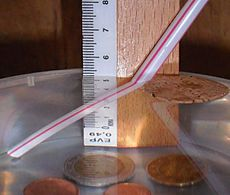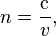# Refraction facts for kids

Kids Encyclopedia Facts
(Redirected from Refractive index)When viewing at a certain angle, the straw appears to bend, due to refraction of light as it moves into the air

Refraction is the change in direction of a wave, caused by the change in the wave's speed. Examples of waves include sound waves and light waves. Refraction is seen most often when a wave passes from one transparent medium to another transparent medium. Different types of medium include air and water.

When a wave passes from one transparent medium to another transparent medium, the wave will change its speed and its direction. For example, when a light wave travels through air and then passes into water, the wave will slow and change direction.

As light goes into a medium which is denser, the light ray will 'bend' toward the normal. When it goes back into the less dense medium (with a lower refractive index), it will bend back through the same angle as when it came in (if the surface at exit is parallel to the surface at entry).

An example of how refraction works is placing a straw in a cup of water, with part of the straw in the water. When looking at a certain angle, the straw appears to bend at the water's surface. This is because of the change in density of the medium and thus, bending of light rays as they move from the air to water.

A good and simple way to understand how light works is to think of it like a car. When the car hits the gravel surface (this is the medium) on an angle, the tire that hits it first will slow, causing it to turn in that direction. Therefore, if light hits a medium on the right having a greater refractive index, it will bend right. The amount of bending is given by Snell's law. Lenses work by refraction.

When light refracts in a prism, it splits into colours of the rainbow because some wavelengths bend more than others.

In optics the refractive index or index of refraction n of a substance is a dimensionless number that describes how light, or other radiation, goes through that medium. It is defined as$n = \frac{\mathrm{c}}{v},$

where c is the speed of light in a vacuum and v is the phase velocity of light in the medium. Snell's law uses refractive indexes to calculate the amount of refraction.

## Images for kidsRefraction Facts for Kids. Kiddle Encyclopedia.